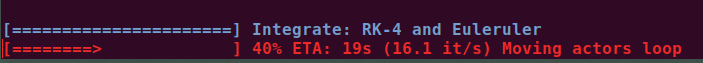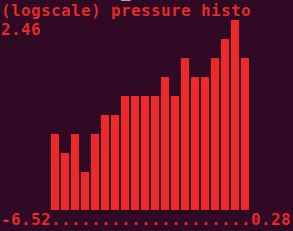# utils¶

Utilities submodule.

## ProgressBar¶

class vedo.utils.ProgressBar(start, stop, step=1, c=None, bold=True, italic=False, title='', ETA=True, width=25, char='━', char_back='─')[source]

Bases: object

Class to print a progress bar with optional text message.

Example
import time
pb = ProgressBar(0,400, c='red')
for i in pb.range():
time.sleep(.1)
pb.print('some message')len()[source]

Return the number of steps.

print(txt='', counts=None, c=None)[source]

Print the progress bar and optional message.

range()[source]

Return the range iterator.

## cameraFromNeuroglancer¶

vedo.utils.cameraFromNeuroglancer(state, zoom=300)[source]

Define a vtkCamera from a neuroglancer state dictionary.

Parameters
• state (dict) – an neuroglancer state dictionary.

• zoom (float) – how much to multiply zoom by to get camera backoff distance default = 300 > ngl_zoom = 1 > 300 nm backoff distance.

Returns

a vtk camera setup that matches this state.

Return type

vtk.vtkCamera

## cameraFromQuaternion¶

vedo.utils.cameraFromQuaternion(pos, quaternion, distance=10000, ngl_correct=True)[source]

Define a vtkCamera with a particular orientation.

Parameters
• pos (np.array, list, tuple) – an iterator of length 3 containing the focus point of the camera

• quaternion (np.array, list, tuple) – a len(4) quaternion (x,y,z,w) describing the rotation of the camera such as returned by neuroglancer x,y,z,w all in [0,1] range

• distance (float) – the desired distance from pos to the camera (default = 10000 nm)

Returns

a vtk camera setup according to these rules.

Return type

vtk.vtkCamera

## cart2pol¶

vedo.utils.cart2pol(x, y)[source]

Cartesian to Polar coordinates conversion.

## cart2spher¶

vedo.utils.cart2spher(x, y, z)[source]

Cartesian to Spherical coordinate conversion.

## dotdict¶

class vedo.utils.dotdict(*args, **kwargs)[source]

Bases: dict

A dictionary supporting dot notation.

Example

dd = dotdict({"a": 1,
"b": {"c": "hello",
"d": [1, 2, {"e": 123}]}
}
)
dd.update({'k':3})
dd.g = 7
print("k=", dd.k)           # k= 3
print(dd.b.c)               # hello
print(isinstance(dd, dict)) # True
print(dd.lookup("b.d"))     # [1, 2, {"e": 123}]

lookup(dotkey)[source]

Lookup value in a nested structure with a single key, e.g. “a.b.c”.

## fitCircle2D¶

vedo.utils.fitCircle2D(points)[source]

Fits a circle through points in the plane, with a very fast non-iterative method.

Returns the tuple (center_x, center_y, radius).

Reference: J.F. Crawford, Nucl. Instr. Meth. 211, 1983, 223-225.

Note

E.g.:

from vedo import *

x = arange(0, 3, 0.1)
y = sin(x)

cx, cy, R = fitCircle2D([x,y])
Points([x, y])
Point([cx, cy])
Circle([cx, cy], r=R)
show(..., axes=8)


## geometry¶

vedo.utils.geometry(obj, extent=None)[source]

Apply the vtkGeometryFilter. This is a general-purpose filter to extract geometry (and associated data) from any type of dataset. This filter also may be used to convert any type of data to polygonal type. The conversion process may be less than satisfactory for some 3D datasets. For example, this filter will extract the outer surface of a volume or structured grid dataset.

Returns a Mesh object.

Parameters

extent (list) – set a [xmin,xmax, ymin,ymax, zmin,zmax] bounding box to clip data.

## grep¶

vedo.utils.grep(filename, tag, firstOccurrence=False)[source]

Greps the line that starts with a specific tag string inside the file.

## humansort¶

vedo.utils.humansort(l)[source]

Sort in place a given list the way humans expect.

NB: input list is modified

E.g. [‘file11’, ‘file1’] -> [‘file1’, ‘file11’]

## isSequence¶

vedo.utils.isSequence(arg)[source]

Check if input is iterable.

## linInterpolate¶

vedo.utils.linInterpolate(x, rangeX, rangeY)[source]

Interpolate linearly variable x in rangeX onto rangeY. If x is a 3D vector the linear weight is the distance to the two 3D rangeX vectors.

E.g. if x runs in rangeX=[x0,x1] and I want it to run in rangeY=[y0,y1] then y = linInterpolate(x, rangeX, rangeY) will interpolate x onto rangeY.

## mag¶

vedo.utils.mag(z)[source]

Get the magnitude of a vector.

## mag2¶

vedo.utils.mag2(z)[source]

Get the squared magnitude of a vector.

## makeBands¶

vedo.utils.makeBands(inputlist, numberOfBands)[source]

Group values of a list into bands of equal value.

Parameters

numberOfBands (int) – number of bands, a positive integer > 2.

Returns

a binned list of the same length as the input.

## orientedCamera¶

vedo.utils.orientedCamera(center=0, 0, 0, upVector=0, 1, 0, backoffVector=0, 0, 1, backoff=1)[source]

Generate a vtkCamera pointed at a specific location, oriented with a given up direction, set to a backoff.

## pointIsInTriangle¶

vedo.utils.pointIsInTriangle(p, p1, p2, p3)[source]

Return True if a point is inside (or above/below) a triangle defined by 3 points in space.

## pointToLineDistance¶

vedo.utils.pointToLineDistance(p, p1, p2)[source]

Compute the distance of a point to a line (not the segment) defined by p1 and p2.

## pol2cart¶

vedo.utils.pol2cart(rho, theta)[source]

Polar to Cartesian coordinates conversion.

## precision¶

vedo.utils.precision(x, p, vrange=None, delimiter='e')[source]

Returns a string representation of x formatted with precision p.

Parameters

vrange (float) – range in which x exists (to snap it to ‘0’ if below precision).

Based on the webkit javascript implementation taken from here, and implemented by randlet.

## printHistogram¶

vedo.utils.printHistogram(data, bins=10, height=10, logscale=False, minbin=0, horizontal=False, char='▉', c=None, bold=True, title='Histogram')[source]

Ascii histogram printing. Input can also be Volume or Mesh. Returns the raw data before binning (useful when passing vtk objects).

Parameters
• bins (int) – number of histogram bins

• height (int) – height of the histogram in character units

• logscale (bool) – use logscale for frequencies

• minbin (int) – ignore bins before minbin

• horizontal (bool) – show histogram horizontally

• char (bool) – character to be used

• c (str,int) – ascii color

• char – use boldface

• title (str) – histogram title

Example
from vedo import printHistogram
import np as np
d = np.random.normal(size=1000)
data = printHistogram(d, c='blue', logscale=True, title='my scalars')
data = printHistogram(d, c=1, horizontal=1)
print(np.mean(data)) # data here is same as d## printInfo¶

vedo.utils.printInfo(obj)[source]

Print information about a vtk object.

## resampleArrays¶

vedo.utils.resampleArrays(source, target, tol=None)[source]

Resample point and cell data of a dataset on points from another dataset. It takes two inputs - source and target, and samples the point and cell values of target onto the point locations of source. The output has the same structure as the source but its point data have the resampled values from target.

Parameters

tol (float) – set the tolerance used to compute whether a point in the target is in a cell of the source. Points without resampled values, and their cells, are be marked as blank.

## spher2cart¶

vedo.utils.spher2cart(rho, theta, phi)[source]

Spherical to Cartesian coordinate conversion.

## trimesh2vedo¶

vedo.utils.trimesh2vedo(inputobj, alphaPerCell=False)[source]

Convert Trimesh object to Mesh(vtkActor) or Assembly object.

## vector¶

vedo.utils.vector(x, y=None, z=0.0, dtype=<class 'numpy.float64'>)[source]

Return a 3D numpy array representing a vector.

If y is None, assume input is in the form [x,y,z].

## vedo2trimesh¶

vedo.utils.vedo2trimesh(mesh)[source]

Convert vedo.Mesh to Trimesh.Mesh object.

## versor¶

vedo.utils.versor(v)[source]

Return the unit vector. Input can be a list of vectors.

## vtkCameraToK3D¶

vedo.utils.vtkCameraToK3D(vtkcam)[source]

Convert a vtkCamera object into a 9-element list to be used by K3D backend.

Output format is: [posx,posy,posz, targetx,targety,targetz, upx,upy,upz]